## Cipher## Apr 28, 2017

### Masonic Arch + 9/11

Everyone that is even remotely familiar with Freemasonic symbolism will immediately recognize the Masonic Arch but there's a very special number showing up when we measure half of the zodiac wheel like this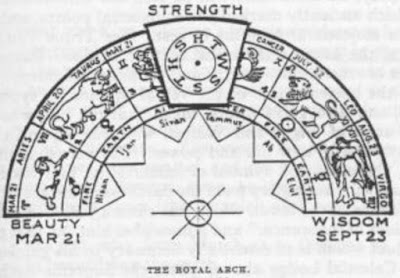From Spring Equinox (3/21) to Autumnal Equinox (9/23) is 187 days, this is one of the extremely significant numbers that seems to appear everywhere (also it is a very special number due to it's properties, factorization - 11x17 = 187 (187th prime number is 1117)

Bible begins with 5 books of Torah (the "law" as it is often translated), also Torah is 187 chapters long
Five books of Torah = 6+9+22+5 + 2+15+15+11+19 + 15+6 + 20+15+18+1+8 = 187 (Ordinal)
Aslo Aleister Crowley made his own ALW (LAW) gematria cipher and if you think about his teachings it's all based around Law.
The order & value of the letters in the ALW Cipher are derived from the grid superimposed on one of the pages of manuscript of Liber AL on which this verse appears (sheet 16 of Chapter III).
English Alphabet = 25+14+11+2+23+5+4 + 1+2+26+4+1+20+25+24 = 187 (ALW)

418 is a number connecting to entity Crowley encountered that is called "Aiwass", on the cover of one of his books you can see XCIII = 418 (93 = 418)
There's a lot more to be said about 187 but I don't wanna get sidetracked too much

So when I started thinking about importance of astrology to these people the first thing I measured from was 9/11 to Spring Equinox and Winter Solstice in 20019/11 attack lasted for 102 minutes, there was a 3/11/2004 Madrid Bombing 911 days after 9/11/2001 and we have 14 weeks and 4 days (144 is a very special number related to Time)
Madrid Train bombings = 4+1+4+9+9+4 + 2+9+1+9+5 + 2+6+4+2+9+5+7+10 = 102 (Single Reduction)One of the "airplanes" that hit the buildings was Flight 175 which was the most mysterious number to me and measuring from Spring Equinox I think we found the answer### Fahrenheit 9/11 documentary

Everything about this documentary is incredibly well calculated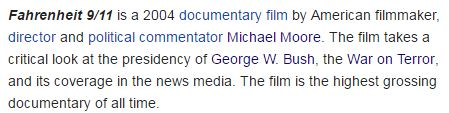They give us 2 release dates for this propaganda piece

5/17/2004 - 5+1+7+2+0+0+4 = 19 (this date could also be written 17/5 a lot like Flight 175)
6/25/2004 - 6+2+5+2+0+0+4 = 19, in 2004 George Bush celebrated his birthday on a date with 19 numerology as well (7 + 6 + 2+0+0+4 = 19)
Including the end date these dates are 1 month and 9 days apart (There were supposedly 19 hijackers on September 11th)Forty = 6+60+90+200+700 = 1056 (English Extended)
Drop the zero from 1056 and you find 911 encoded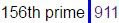From 9/11/2001 to 6/25/2004 is 1019 days - Twenty fifth of June = 20+23+5+14+20+25 + 6+9+6+20+8 + 15+6 + 10+21+14+5 = 227 (English Ordinal)If we measure from 5/17/2004 to Bush's next birthday it gives us 1 month and 19 days - 50 days - Fahrenheit = 3+8+1+9+4+4+1+4+9+7 = 50 (Reverse Reduction)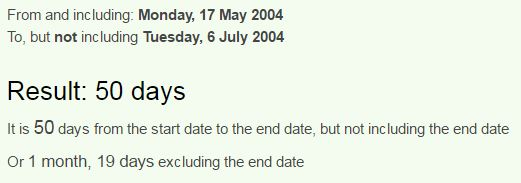Also if we measure from Bush's birthday in 2003 we find 11 months and 19 days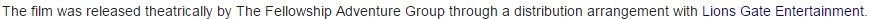Lionsgate = 20+9+50+40+90+7+1+100+5 = 322 (Jewish)
George Bush is born on "Sixth of July" = 100+9+600+200+8 + 60+6 + 10+300+30+700 = 2023 (English Extended)

Lionsgate entertainment = 3+9+6+5+10+7+1+2+5 + 5+5+2+5+9+2+1+9+5+4+5+5+2 = 107 (Single Reduction)
George Bush = 7+5+15+18+7+5 + 2+21+19+8 = 107 (English Ordinal)
Bush declared War on Afghanistan on 10/7/2001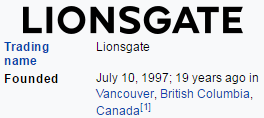Even Lionsgate was founded on a date that would be written 10/7 in most parts of the world.Everywhere they say that Bush spent about 20 minutes in the classroom - "twenty" = 20+23+5+14+20+25 = 107 (English Ordinal)

Documentary was produced by Michael = 4+9+3+8+1+5+3 = 3(Full Reduction)Moore = 13+15+15+18+5 = 66 (English Ordinal)
Thirty three = 2+8+9+9+2+7 + 2+8+9+5+5 = 66 (Full Reduction)

"Michael Moore" = 13+9+3+8+1+5+12 + 13+15+15+18+5 = 117 (English Ordinal)Bush became 46th governor of Texas on 1/17 and he won the presidential election on 11/7/2000 exactly 303 weeks later (When you say September eleventh you are really saying 7/11)I can get into a lot more stuff but a lot of it is gonna be covered in Derek's 9/11 video that's coming soon

## Apr 27, 2017

### CNN - Airline passengers caught with 10 kilos of cocaine in pants... absolute joke

Today is April 27th and here is another hilarious story that took place on April 19th... I don't know how they can get away with these stories but anyone that ever got on a plane would realize that you would never get away with taping cocaine to your legs...

ten kilograms = 20+5+14 + 11+9+12+15+7+18+1+13+19 = 144 (English Ordinal)
Note at what Time the story was updatedAs always they give us something nice in the title (7x7x7 = 343)April 19th was a date with 33 numerology (4+19+ 2+0+1+7 = 33)
They tried to smuggle 10 kilos of cocaine
smuggle = 8+5+6+2+2+6+4 = 33 (Reverse Reduction)
ten kilos = 2+5+5 + 2+9+3+6+1 = 33 (Full Reduction)
In the statement, it was not mentioned what tipped off the officers to conduct additional inspections on the men. But they were taken to a private search room where officers seized 23 pounds (10.4 kilos) of cocaine estimated at a nearly \$400,000 street value.
twenty three pounds = 2+5+5+5+2+7 + 2+8+9+5+5 + 7+6+3+5+4+10 = 90 (Single Reduction)
ninety = 5+9+5+5+2+7 = 33 (Full Reduction)
Also they are saying that it's nearly worth 400,000 dollars in street value33rd prime number is 137

They took 23 pounds on 4/19 = 4+19 = 23, the story is coming out on CNN today on a date with 23 numerology (4+2+7+2+0+1+7 = 23)
cocaine = 3+6+3+1+9+5+5 = 32 (Full Reduction)

cocaine = 6+3+6+8+9+4+4 = 40 (Reverse Reduction), they were caught on JFK airport located at 40°North latitude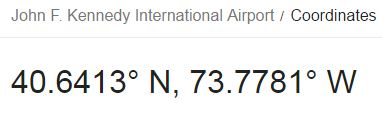Last month at the same airport, officers said they discovered two other passengers on separate days with drugs taped under their pants.The last time they reported something like this was on March 17th and this stupid story is released on the 41st day from March 17th on a date with 41 numerology (4+27 + 2+0+1+7  = 41)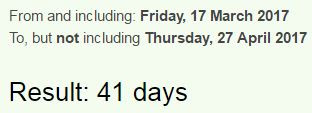If we don't count the end date it's a 40 day span connecting to cocaine = 6+3+6+8+9+4+4 = 40 (Reverse Reduction)

The guys that were caught on the 19th traveled on the same flight from "Dominican Republic to New York" = 4+15+13+9+14+9+3+1+14 + 18+5+16+21+2+12+9+3 + 20+15 + 14+5+23 + 25+15+18+11 = 314 (English Ordinal)

## Apr 26, 2017

### CNN - 3 foot long giant rabbit died on United Airlines flight?

http://edition.cnn.com/2017/04/26/travel/bunny-death-united-trnd/index.html
Earlier today I was checking CNN and I noted that they published a story on Dr. David Dao once again, so I was wondering why they were bringing it up today and this seems to be the reason, today they are talking about a giant 3 foot rabbit that died after United Airlines flight, this has to be at least 3rd or 4th time that something is happening on United Airlines flight in the last 2 months and it's really getting hilarious at this pointYou know it's a really good story when they open with 911 coded into the headline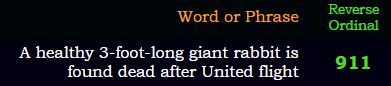United Airlines planes were supposedly used for the 9/11 attackThe rabbit was flying from "Heathrow Airport to O'Hare" = 1+4+8+7+1+9+3+4 + 8+9+9+2+3+9+7 + 7+3 + 3 + 1+8+9+4 = 119 (Reverse Reduction)
three foot long giant rabbit = 2+8+9+5+5 + 6+6+6+2 + 3+6+5+7 + 7+9+1+5+2 + 9+1+2+2+9+2 = 119 (Full Reduction)

Rabbit Simon died on April 19th on a date with 33 numerology (4 + 19 +2+0+1+7 = 33) and the story is coming 7 days later- one week = 6+5+5 + 5+5+5+2 = 33 (Full Reduction)"seven days" = 1+5+4+5+5 + 4+1+7+1 = 33 (Full Reduction)Darius = 23+26+9+18+6+8 = 90 (Reverse Ordinal)
Ninety = 5+9+5+5+2+7 = 33 (Full Reduction)April = 8+2+9+9+6 = 34 (Reverse Reduction)
Rabbit = 90+1+2+2+9+200 = 304 (English Extended)
Simon = 10+9+4+6+5 = 34 (Single Reduction)Note how their emphasize "Saddened" = 8+8+5+5+4+4+4+5 = 43 (Reverse Reduction)
Also "saddened" and Simon have matching gematria
"Simon" = 8+9+5+3+4 = 29 (Reverse Reduction)
"Saddened" = 1+1+4+4+5+5+5+4 = 29 (Full Reduction)

I have no clue what they're trying to accomplish with these stories and United Airlines..

## Apr 22, 2017

### CNN: Venezuela protests: Looting, shootings leave 13 dead in one day - 2014 protest connection

I don't have time to decode this story so I'll just give you the best thing right awayVenezuelan protests started in 2014 and they tried to have an interesting tribute to the start of the protest.
Look at the title, it starts off with - Venezuela protests = 5+22+13+22+1+6+22+15+26 + 11+9+12+7+22+8+7+8 = 216 (Reverse Ordinal)
The second part of the headline is way more interesting157 is half of 314, also 364 could go in with the circle and time symbolism, but also we have 2014 telling us to measure from the start of the protest basicallyFrom the first day of protest to the release of this story is exactly 1200 days (3 years, 3 months, 14 days)https://en.wikipedia.org/wiki/Timeline_of_the_2014_Venezuelan_protests

Here we see that protests started on January 8th, after the killing of Monica Spear
Monica Spear = 5+3+4+9+6+8 + 8+2+4+8+9 = 66 (Reverse Reduction)
The protests are taking place in Caracas located at 66°West
January Eighth = 1+1+5+3+1+9+7 + 5+9+7+8+2+8 = 66 (Full Reduction)

She was killed on a 1/6/2014 a date with 41 numerology (13th prime number is 41), and today they are taking about 13 dead in one day
1/6/2014 - 1+6+20+14 = 41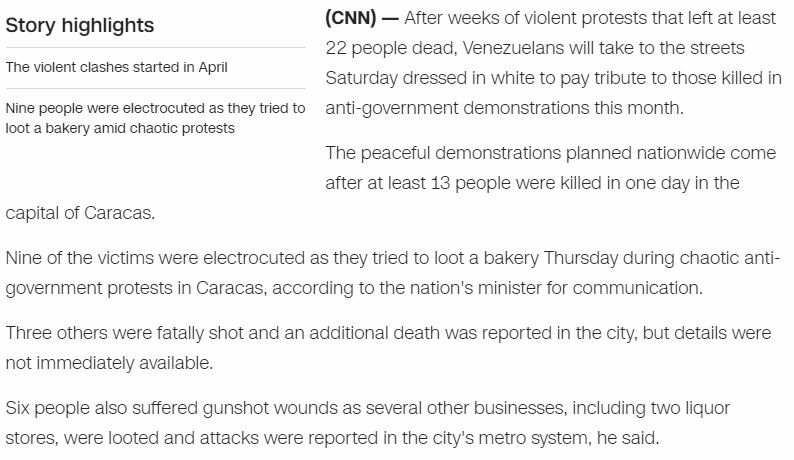## Apr 18, 2017

### Terence McKenna murder by the numbers... 227/318

This is gonna be a short post on the murder of Terence McKenna... I honestly think there's no chance that he died from a "rare form of brain cancer"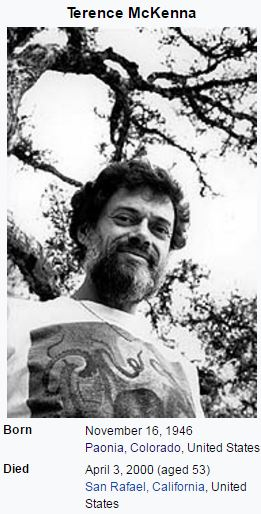Terence McKenna died on April 3rd, 93rd day and a date coming 227 days before his birthday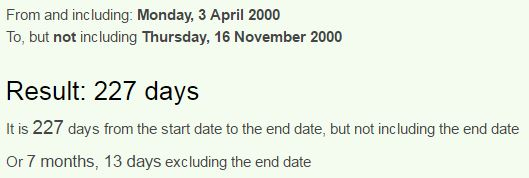Also note 7 months 13 days... a lot like 713 or the reflection 317 (66th prime number)
Dennis McKenna = 4+5+40+40+9+90 + 30+3+10+5+40+40+1 = 317 (Jewish)Note that his brother is born on the first day of ancient Roman Holiday called Saturnalia, this is very interesting because Terence died on 93rd day of the year, Saturn = 93 (English Ordinal), Terence =  119 (Reverse Ordinal), Saturn = 45+1+20+21+18+14 = 119 (Francis Bacon)
From the birthday of his brother in 1999 to his death is 3 months and 18 days, connecting back to Pi (22/7 = 3.14, 7/22 = 0.318....)McKenna died on a date with 27 numerology (4+3+20+00 = 27) and this is very interesting considering his Library burned down 7 years after his death on February 7th, written 2/7 in the USNote that this came 314 days before birthday of his brother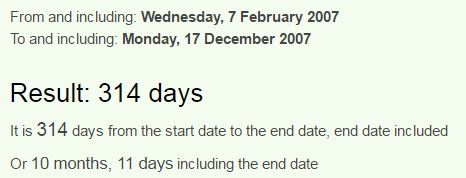February 7th is the 38th day of the year
Terence = 7+4+9+4+4+6+4 = 38 (Reverse Reduction)
McKenna = 5+6+7+4+4+4+8 = 38 (Reverse Reduction)
This library fire took place 1 month and 28 days before anniversary of his death, connecting to his last name...McKenna = 14+24+16+22+13+13+26 = 128 (Reverse Ordinal)

## Apr 17, 2017

### WWE - The perfect sacrifice - Curt Hennig - 227/119

Quick post on sacrifice of Curt Hennig / Mr. Perfect - the people writing these scripts are 100% insane...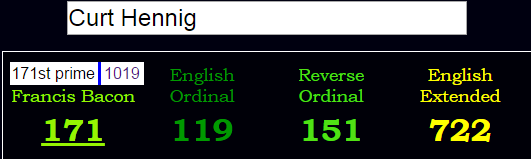His name gematria is completely insane (151 is 227 in octal numbering system - 22/7 = 3.14) -Mister = 30+9+90+100+5+80 = 314 (Jewish) Mr. Perfect = 5+9 + 2+4+9+3+4+6+7 = 49 (Reverse Reduction)Note that he died from "Acute drug intoxication" = 26+24+6+7+22 + 23+9+6+20 + 18+13+7+12+3+18+24+26+7+18+12+13 = 314 (Reverse Ordinal)

He died 1 month and 19 days before his birthday on 28th of MarchEven his stage name has a connection to 911 - Mr. Perfect = 39+18 + 42+5+18+6+5+3+20 = 156 (Francis Bacon)Curt fought for the last time on 1/8/2003 which is 9 months and 11 days after his birthday..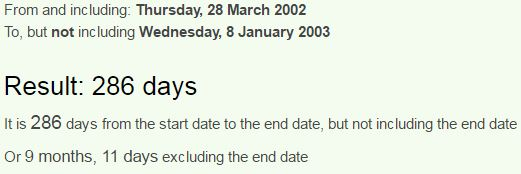His father was also a professional wrestler for AWA - Larry "The Axe" Hennig = 20+1+80+80+400 + 100+8+5 + 1+300+5 + 8+5+40+40+9+7 = 1109 (Jewish)
Larry = 30+1+90+90+700 = 911 (English Extended)His father was born on June eighteenth which also connects to PiCurt died 4 months and 9 days (49th prime is 227) before his fathers birthday in 2003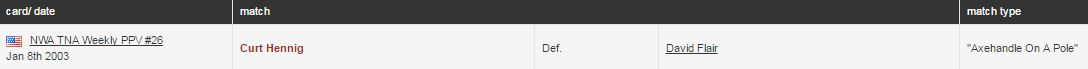First I want you to note this match type: "Axehandle on a pole" - his father went by "The Axe"

Curt had his last match on 1/8/2003 against David Flair, and just before it started he said something extremely interesting: "I've got a saying in the wrestling business, and it goes like this: Paying your dues in the wrestling business... 141 million. Being the greatest athlete in sports entertainment.. Another 67 million. Taking down Brock Lesnar at 35,000 feet? Priceless!!
https://www.youtube.com/watch?v=Csitehtm8UI - here you can watch his last speech/fight

He says 141 million and 67 million before the match started... (his opponent had name gematria of 67 - David Flair = 5+8+5+9+5 + 3+6+8+9+9 = 67 (Reverse Reduction), if you watch the video you will see that Flair struck Curt with a "Burlap sack" = 67 (Reverse Reduction)
"Mister Perfect" = 4+9+10+2+5+9 + 7+5+9+6+5+3+2 = 76 (Single Reduction)

Just look at the weight of David Flair and you see another Pi connection, it might actually be the reason he was chosen for the match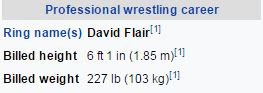Curt Hennig had a total of 448 matches in his wrestling career which even connects to 314 through base-12 numbering system....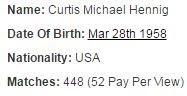Moving on to the second number he mentioned which has far more interesting connections here, "Four hundred forty eight matches" = 3+3+6+9 + 1+6+4+5+9+4+5 + 3+3+9+7+2 + 4+9+2+1+7 + 5+8+7+6+1+4+8 = 141 (Reverse Reduction)
"World Championship Wrestling" = 5+6+9+3+4 + 3+8+1+4+7+9+6+5+1+8+9+7 + 5+9+5+1+2+3+9+5+7 = 141 (Full Reduction)
He died 33 days after his last match - Thirty three = 7+19+18+9+7+2 + 7+19+9+22+22 = 141 (Reverse Ordinal) - note that his middle name is "Michael" = 4+9+3+8+1+5+3 = 33 (Full Reduction), the name of his theme song is "Perfection" = 42+5+18+6+5+3+20+9+15+14 = 137 (Francis Bacon)So at the end of the speech Curt mentions his airplane fight with Brock Lesnar which is very important part of the speech and whoever wrote it is very much into gematria

It was the 5th May 2002, and the WWE crew was flying back from Britain to America. Perhaps the most dangerous aspect of the flight was the fight between Curt Henning and Brock Lesnar. Mr Perfect had started joking he was a better wrestler than Brock, so the two had a play wrestle right there in the plane aisle. This soon got a bit too serious, and at one point Lesnar smashed Hennig against the plane walls so hard that the flight attendants thought the plane was going to break open. Henning was released soon after.

After the airplane fight on 5/5/2002 he had a match the next day and they released him a few days later,  then he came back to fight 156 days later on October 9th and he died after the 9th match from his return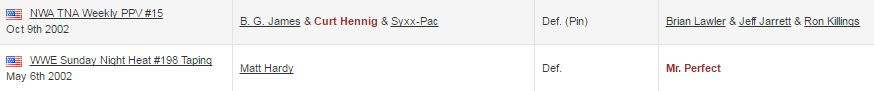Taking down Brock Lesnar at 35,000 feet? Priceless!!
"thirty five thousand feet" = 7+19+18+9+7+2 + 21+18+5+22 + 7+19+12+6+8+26+13+23 + 21+22+22+7 = 314 (Reverse Ordinal)

Now here is the interesting part - "Brock Lesnar" = 2+18+15+3+11 + 12+5+19+14+1+18 = 118 (English Ordinal)
"thirty five thousand feet" = 2+8+9+9+2+7 + 6+9+4+5 + 2+8+6+3+10+1+5+4 + 6+5+5+2 = 118 (Single Reduction)
Excluding the end date we see that he died 46 days (1 month and 18 days) before his birthday - Brock Lesnar = 2+9+6+3+2 + 3+5+1+5+1+9 = 46 (Full Reduction)"Death" = 4+5+1+100+8 = 118 (Jewish)
Even 22/7 connects to 118... "Twenty two divided by seven" = 7+4+4+4+7+2 + 7+4+3 + 5+9+5+9+5+4+5 + 7+2 + 8+4+5+4+4 = 118 (Reverse Reduction)
Curt Hennig died on 2/10 which is the 41st day of the year - "Forty one" = 6+15+18+20+25 + 15+14+5 = 118 (English Ordinal)

In the image below you see what Brock Lesnar wrote about Curt in his autobiographical book called DeathClutch, I find the first part very interesting where he talks about paying the price to achieve success in wrestling business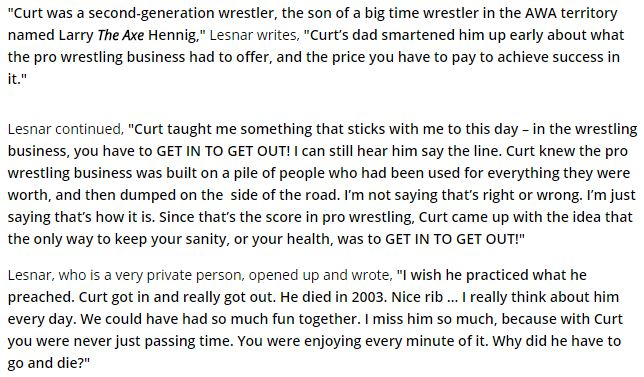From the airplane fight on 5/5/2002 to his next birthday (3/28/2003) is 328 days...He died at the age of 44 on a date with 17 numerology (2+10 + 2+0+0+3 = 17)
Kill = 44/17
Execution = 44

There are a few interesting numbers coming up in his career but most of them are unrelated to his death so I'll just end the post here. I think this is good enough proof of this death being very calculated in advance